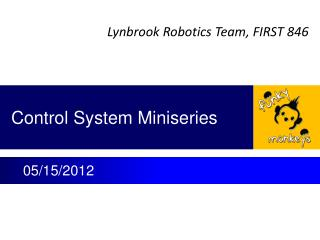DownloadDownload PresentationControl System Miniseries

# Control System Miniseries

Download Presentation## Control System Miniseries

- - - - - - - - - - - - - - - - - - - - - - - - - - - E N D - - - - - - - - - - - - - - - - - - - - - - - - - - -
##### Presentation Transcript

1. Control System Miniseries 05/15/2012

2. Lecture 1 • What is control system • Control system diagram and terminology • Mathematic relations.

3. What Is Control System • A control system is a device, or set of devices to manage, command, direct or regulate the behavior of other devices or system. – From Wikipedia. • Feed forward (open loop) control • Shooter tester • Feedback (close loop) control • Shooter with hall effect speed sensor

4. Feed Forward Control Control Variable Controller Plant Input Output Xctrl C P Xcmd Xout In time domain: Xctrl (t) = C(Xcmd, t) Xout(t) = P(Xctrl, t), For a linear system and in frequency domain: Xctrl (s) = C(s)Xcmd(s) Xout(s) = P(s) Xctrl(s) = P(s) C(s) Xcmd(s) s = jω = j(2πf), f - frequency Plant – a physical system to be controlled; robot driving base, shooter wheel Input – Target/desired value of plant output; robot position, shooter speed, joystick input counts Controller – signal converter, conditioner; Control Variables – Physical signal which plant can take as input; voltage, torque Output – Physical response of plant to its input.

5. Feedback Control System Control Variable Input Error + Controller Plant Output - Feedback Sensor Xcmd Err Xctrl + C P Xout - Xfbk S In time domain: Xfbk(t) = S(Xout, t) Err(t) = Xcmd(t) – Xfbk(t) Xctrl (t) = C(Err, t) Xout(t) = P(Xctrl, t), For a linear system and in frequency domain: Xfbk(s) = S(s) Xout (s) E(s) = Xcmd(s) – Xfbk(s) Xctrl (s) = C(s) E(s) = C(s) [Xcmd(s) - Xout(s)] Xout(s) = P(s) Xctrl(s) = P(s) C(s) [Xcmd(s) - Xout(s)] Xout(s) = {P(s)C(s) /[1 + P(s) C(s) S(s)]} Xcmd(s)

6. Example Motor Output Torque Gearbox Output Torque Calculated Wheel Speed Speed Error Control Voltage Motor Voltage Wheel Speed Δω (rpm) Vctrl (volt) Vm (volt) Tm (N-m) Tgb (N-m) ωwhl (rpm) ω0 (rpm) + Control Software Jaguar Speed Controller Motor Gearbox Shooter Wheel - Controller Plant Sensor Voltage to Speed Converter Hall Effect Sensor (Voltage Pulse Generator Pulse Counter ωfbk (rpm) Vpls (volt) Pwhl (# of pulse) Measured Wheel Speed Voltage of Pulse Rate Sensor Pulse • Present every major component • Label variables and physical unit • Label conversion factor

7. Summary • Major control system include • plant, controller and sensor blocks • Each block contains many physical components. • Present physical components with input and output variables. • Establish math function between input and output of each component with proper unit.

8. Homework (two weeks) • Find the conversion factors for each box of shooter control diagram (analytical, experimental, or online search). • Write done the algorithm (logic steps) of current version of control SW. • Advanced home work • What is block diagram (mathematic relation) of a motor? • Is Jaguar a simple proportional components? • Besides driving torque, are there any other torques acting on shooter wheel?

9. Make a Test Fixture for Lecture 2 • Find a spring and weight • Make resonator about a couple to a few Hertz. • Make different thickness (viscosity) liquid out of powder material. • Dip weight ball or a rod into liquid. • Suddenly lift top end of spring a distance. • Observe weight movement. Spring Indicator Container Weight (ball) Thinner liquid Thicker liquid